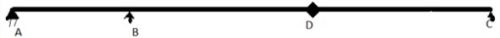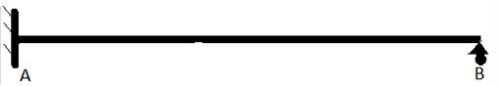Courses

# Test: Influence Lines For Statically Indeterminate Beams - 1

## 10 Questions MCQ Test Structural Analysis | Test: Influence Lines For Statically Indeterminate Beams - 1

Description
This mock test of Test: Influence Lines For Statically Indeterminate Beams - 1 for GATE helps you for every GATE entrance exam. This contains 10 Multiple Choice Questions for GATE Test: Influence Lines For Statically Indeterminate Beams - 1 (mcq) to study with solutions a complete question bank. The solved questions answers in this Test: Influence Lines For Statically Indeterminate Beams - 1 quiz give you a good mix of easy questions and tough questions. GATE students definitely take this Test: Influence Lines For Statically Indeterminate Beams - 1 exercise for a better result in the exam. You can find other Test: Influence Lines For Statically Indeterminate Beams - 1 extra questions, long questions & short questions for GATE on EduRev as well by searching above.
QUESTION: 1

### Ray = reaction at support A in y direction. Sign convention is as usual. Beam is linear elastic. Point A is a pin joint and point B and C are roller support. D is just an arbitrary point. Unit load is applied at point D.Q. What will be the value of Ray in the above figure?

Solution:

Explanation: If we remove the support at point A and replace it by force Faa. Due to sign convention, its direction will be initially considered upward.

QUESTION: 2

### Ray = reaction at support A in y direction. Sign convention is as usual. Beam is linear elastic. Point A is a pin joint and point B and C are roller support. D is just an arbitrary point. Unit load is applied at point D.Q. What will be the difference between magnitudes of Fad and Fda?

Solution:

Explanation: Due to validity of Maxwell’s reciprocal theorem, magnitudes of Fad and Fda will be equal.

QUESTION: 3

### Ray = reaction at support A in y direction. Sign convention is as usual. Beam is linear elastic. Point A is a pin joint and point B and C are roller support. D is just an arbitrary point. Unit load is applied at point D.Q. What is the relation between Fad and Fda?

Solution:

Explanation: Magnitudes will be same as stated above. Now, sign will be opposite as slope at B won’t change in deflection diagram and there won’t be and vertical deflection at point C.

QUESTION: 4

Ray = reaction at support A in y direction.
Sign convention is as usual.
Beam is linear elastic.
Point A is a pin joint and point B and C are roller support. D is just an arbitrary point.
Unit load is applied at point D.Q. Which of the following is equal to Ray?

Solution:

Explanation: Just replacing the value of Fad in the answer of Q1 will give us the result. This relation will be the basic equation for ILD of indeterminate structures.

QUESTION: 5

Ray = reaction at support A in y direction.
Sign convention is as usual.
Beam is linear elastic.
Point A is a pin joint and point B and C are roller support. D is just an arbitrary point.
Unit load is applied at point D.Q. The relationship between Ray,Fda and Faa is valid only for point A.
State whether the above statement is true or false.

Solution:

Explanation: It is valid for all supports like B and C, just replace all a’s by that point. We haven’t used any support conditions of point A while deriving this equation.

QUESTION: 6

A is a fixed support and B is roller.
AB = 18m.
C is a point at a distance of 6m from point A.
D is a point at a distance of 6m from point B.
Assume E and I to be given.What is the DSI for this structure?

Solution:

Explanation: Total 4 unknowns are there and 3 equations are there.

QUESTION: 7

A is a fixed support and B is roller.
AB = 18m.
C is a point at a distance of 6m from point A.
D is a point at a distance of 6m from point B.
Assume E and I to be given.Q. How many redundant forces are there in the above structure?

Solution:

Explanation: 1 redundant force is there as it is equal to DSI.
We have taken the horizontal force at point A as redundant force.

QUESTION: 8

A is a fixed support and B is roller.
AB = 18m.
C is a point at a distance of 6m from point A.
D is a point at a distance of 6m from point B.
Assume E and I to be given.Q.  Which support should replace fixed support at point A for above condition?

Solution:

Explanation: Only slider will give both moment and vertical reaction.

QUESTION: 9

A is a fixed support and B is roller.
AB = 18m.
C is a point at a distance of 6m from point A.
D is a point at a distance of 6m from point B.
Assume E and I to be given.Q. What will be the value of Ray for ILD?

Solution:

Explanation: Since redundant force is applied at point A, Fda/Faa will be the result unity.

QUESTION: 10

A is a fixed support and B is roller.
AB = 18m.
C is a point at a distance of 6m from point A.
D is a point at a distance of 6m from point B.
Assume E and I to be given.Q. What will be the value of Δaa?

Solution: